# MAFS.8.EE.1.1Archived StandardExport Print
Know and apply the properties of integer exponents to generate equivalent numerical expressions. For example, 3² ×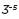=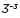=1/3³=1/27.
General Information
Subject Area: Mathematics
Domain-Subdomain: Expressions & Equations
Cluster: Level 1: Recall
Cluster: Work with radicals and integer exponents. (Major Cluster) -

Clusters should not be sorted from Major to Supporting and then taught in that order. To do so would strip the coherence of the mathematical ideas and miss the opportunity to enhance the major work of the grade with the supporting clusters.

Date of Last Rating: 02/14
Status: State Board Approved - Archived
Assessed: Yes
Test Item Specifications

• Assessment Limits :
s Exponents must be integers. Bases must be whole numbers. Variables may not be used.
• Calculator :

No

• Context :

No context

Sample Test Items (3)
• Test Item #: Sample Item 1
• Question:

Which expression is equivalent to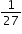?

• Difficulty: N/A
• Type: MC: Multiple Choice

• Test Item #: Sample Item 2
• Question:

Which expression is equivalent to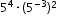?

• Difficulty: N/A
• Type: MC: Multiple Choice

• Test Item #: Sample Item 3
• Question:

An equation is shown.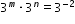What are possible values for m and n?

• Difficulty: N/A
• Type: EE: Equation Editor

## Related Courses

This benchmark is part of these courses.
1205050: M/J Accelerated Mathematics Grade 7 (Specifically in versions: 2014 - 2015, 2015 - 2020, 2020 - 2022, 2022 and beyond (current))
1205070: M/J Grade 8 Pre-Algebra (Specifically in versions: 2014 - 2015, 2015 - 2022, 2022 and beyond (current))
1204000: M/J Foundational Skills in Mathematics 6-8 (Specifically in versions: 2014 - 2015, 2015 - 2022, 2022 and beyond (current))
1200410: Mathematics for College Success (Specifically in versions: 2014 - 2015, 2015 - 2022 (course terminated))
1200700: Mathematics for College Algebra (Specifically in versions: 2014 - 2015, 2015 - 2022 (course terminated))
7812030: Access M/J Grade 8 Pre-Algebra (Specifically in versions: 2014 - 2015, 2015 - 2018, 2018 - 2019, 2019 - 2022, 2022 and beyond (current))
7912115: Fundamental Explorations in Mathematics 2 (Specifically in versions: 2013 - 2015, 2015 - 2017 (course terminated))

## Related Access Points

Alternate version of this benchmark for students with significant cognitive disabilities.

## Related Resources

Vetted resources educators can use to teach the concepts and skills in this benchmark.

## Formative Assessments

Equivalent Powers Expressions:

Students are given numerical expressions and asked to use properties of integer exponents to find equivalent expressions.

Type: Formative Assessment

Exponents Tabled:

Students are asked to complete a table of powers of three and provide an explanation of zero powers.

Type: Formative Assessment

Multiplying and Dividing Integer Exponents:

Students are asked to apply the properties of integer exponents to generate equivalent numerical expressions.

Type: Formative Assessment

Negative Exponential Expressions:

Students are given expressions with negative exponents and are asked to identify those that are equivalent from given sets of expressions.

Type: Formative Assessment

## Lesson Plans

Estimating Length Using Scientific Notation:

This lesson unit is intended to help you assess how well students are able to estimate lengths of everyday objects, convert between decimal and scientific notation and make comparisons of the size of numbers expressed in both decimal and scientific notation.

Type: Lesson Plan

Multiplying terms that have the same base:

Students explore numerical examples involving multiplying exponential terms that have the same base. They generalize the property of exponents where when multiplying terms with the same base, the base stays the same and the exponents are added.

Type: Lesson Plan

Operating with Exponents!:

Students will participate in a gallery walk in which they observe patterns in algebraic expressions. Students will apply the properties of integer exponents to simplify expressions.

Type: Lesson Plan

Stand Up for Negative Exponents:

This low-tech lesson will have students stand up holding different exponent cards to help students write and justify an equivalent expression and see the pattern for expressions with the same base and descending exponents. What happens as you change from 2 to the fourth power to 2 to the third power; 2 to the second power; and so forth? This is an introductory lesson to two of the properties of exponents: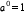and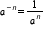Type: Lesson Plan

Scavenger Hunt for Multiplying and Dividing Powers:

Get your students up and moving and interested in simplifying expressions with whole integer powers. After getting your students to figure out what it takes to multiply and divide powers with whole number exponents, have your students scurrying about the room to find the questions and answers for scavenger hunt exercise. The lesson includes questions and answers for the hunt, directions for the hunt, printable cards for the hunt, and step by step directions on how to get your students to figure out what they need to do when multiplying and dividing powers with whole number exponents.

Type: Lesson Plan

Math Is Exponetially Fun!:

The students will informally learn the rules for exponents: product of powers, powers of powers, zero and negative exponents. The activities provide the teacher with a progression of steps that help lead students to determine results without knowing the rules formally. The closing activity is hands-on to help reinforce all rules.

Type: Lesson Plan

Multiplying with Common Bases:

This resource provides a Lesson Plan for teaching students how to apply the Product of Powers Property of exponents. They will be able to write simpler equivalent exponential expressions that are easier to work with and to evaluate when possible.

Type: Lesson Plan

Exponential Chips:

In this lesson students will learn the properties of integer exponents and how to apply them to multiplication and division. Students will have the opportunity to work with concrete manipulatives to create an understanding of these properties and then apply them abstractly. The students will also develop the understanding of the value of any integer to the zero exponent.

Type: Lesson Plan

Ponzi Scheme Pondering:

The students must rationalize how a Ponzi email could make money or fall apart. Using their knowledge of exponential growth, the students can estimate the potential gains, but then are asked to think about why these schemes are illegal and tend to collapse.

Ants versus humans:

This task requires students to work with very large and small values expressed both in scientific notation and in decimal notation (standard form). In addition, students need to convert units of mass. The solution below converts the mass of humans into grams; however, we could just as easily converted the mass of ants into kilograms. Students are unable to go directly to a calculator without taking into account all of the considerations mentioned above. Even after converting units and decimals to scientific notation, students should be encouraged to use the structure of scientific notation to regroup the products by extending the properties of operations and then use the properties of exponents to more fluently perform the calculations involved rather than rely heavily on a calculator.

Extending the Definitions of Exponents, Variation 1:

This is an instructional task meant to generate a conversation around the meaning of negative integer exponents. While it may be unfamiliar to some students, it is good for them to learn the convention that negative time is simply any time before t=0.

## Student Center Activity

Students can practice answering mathematics questions on a variety of topics. With an account, students can save their work and send it to their teacher when complete.

Type: Student Center Activity

## Tutorials

Limits and Infinity:

We will look at examples of limits at infinity.

Type: Tutorial

Exponent Properties Involving Products:

This video discusses exponent properties involving products.

Type: Tutorial

Exponent Properties Involving Quotients:

This video models how to use the Quotient of Powers property.

Type: Tutorial

Negative exponents:

This tutorial shows students the rule for negative exponents. Students will see, using variables, the pattern for negative exponents.

Type: Tutorial

Negative exponents:

In this tutorial, students will learn about negative exponents. An emphasis is placed on multiplying by the reciprocal of a number.

Type: Tutorial

Exponents with Negative Bases:

In this tutorial, you will apply what you know about multiplying negative numbers to determine how negative bases with exponents are affected and what patterns develop.

Type: Tutorial

Exponents and Powers:

This tutorial reviews the concept of exponents and powers and includes how to evaluate powers with negative signs.

Type: Tutorial

Power of a Power Property:

This tutorial demonstrates how to use the power of a power property with both numerals and variables.

Type: Tutorial

Raising Exponential Expressions to Powers:

If a term raised to a power is enclosed in parentheses and then raised to another power, this expression can be simplified using the rules of multiplying exponents.

Type: Tutorial

Raising Products and Quotients to Powers:

Any expression consisting of multiplied and divide terms can be enclosed in parentheses and raised to a power. This can then be simplified using the rules for multiplying exponents.

Type: Tutorial

## Video/Audio/Animations

Exponentiation:

Exponentiation can be understood in terms of repeated multiplication much like multiplication can be understood in terms of repeated addition. Properties of multiplication and division of exponential expressions with the same base are derived.

Type: Video/Audio/Animation

Exponents of One, Zero, and Negative:

Integer exponents greater than one represent the number of copies of the base which are multiplied together. hat if the exponent is one, zero, or negative? Using the rules of adding and subtracting exponents, we can see what the meaning must be.

Type: Video/Audio/Animation

Simplifying Multiplied Exponential Expressions:

Exponential expressions with multiplied terms can be simplified using the rules for adding exponents.

Type: Video/Audio/Animation

Simplifying Divided Exponential Expressions:

Exponential expressions with divided terms can be simplified using the rules for subtracting exponents.

Type: Video/Audio/Animation

Simplifying Mixed Exponential Expressions:

Exponential expressions with multiplied and divided terms can be simplified using the rules of adding and subtracting exponents.

Type: Video/Audio/Animation

## MFAS Formative Assessments

Equivalent Powers Expressions:

Students are given numerical expressions and asked to use properties of integer exponents to find equivalent expressions.

Exponents Tabled:

Students are asked to complete a table of powers of three and provide an explanation of zero powers.

Multiplying and Dividing Integer Exponents:

Students are asked to apply the properties of integer exponents to generate equivalent numerical expressions.

Negative Exponential Expressions:

Students are given expressions with negative exponents and are asked to identify those that are equivalent from given sets of expressions.

## Student Resources

Vetted resources students can use to learn the concepts and skills in this benchmark.

Extending the Definitions of Exponents, Variation 1:

This is an instructional task meant to generate a conversation around the meaning of negative integer exponents. While it may be unfamiliar to some students, it is good for them to learn the convention that negative time is simply any time before t=0.

## Student Center Activity

Students can practice answering mathematics questions on a variety of topics. With an account, students can save their work and send it to their teacher when complete.

Type: Student Center Activity

## Tutorials

Limits and Infinity:

We will look at examples of limits at infinity.

Type: Tutorial

Exponent Properties Involving Products:

This video discusses exponent properties involving products.

Type: Tutorial

Exponent Properties Involving Quotients:

This video models how to use the Quotient of Powers property.

Type: Tutorial

Negative exponents:

This tutorial shows students the rule for negative exponents. Students will see, using variables, the pattern for negative exponents.

Type: Tutorial

Negative exponents:

In this tutorial, students will learn about negative exponents. An emphasis is placed on multiplying by the reciprocal of a number.

Type: Tutorial

Exponents with Negative Bases:

In this tutorial, you will apply what you know about multiplying negative numbers to determine how negative bases with exponents are affected and what patterns develop.

Type: Tutorial

Exponents and Powers:

This tutorial reviews the concept of exponents and powers and includes how to evaluate powers with negative signs.

Type: Tutorial

Power of a Power Property:

This tutorial demonstrates how to use the power of a power property with both numerals and variables.

Type: Tutorial

Raising Exponential Expressions to Powers:

If a term raised to a power is enclosed in parentheses and then raised to another power, this expression can be simplified using the rules of multiplying exponents.

Type: Tutorial

Raising Products and Quotients to Powers:

Any expression consisting of multiplied and divide terms can be enclosed in parentheses and raised to a power. This can then be simplified using the rules for multiplying exponents.

Type: Tutorial

## Video/Audio/Animations

Exponentiation:

Exponentiation can be understood in terms of repeated multiplication much like multiplication can be understood in terms of repeated addition. Properties of multiplication and division of exponential expressions with the same base are derived.

Type: Video/Audio/Animation

Exponents of One, Zero, and Negative:

Integer exponents greater than one represent the number of copies of the base which are multiplied together. hat if the exponent is one, zero, or negative? Using the rules of adding and subtracting exponents, we can see what the meaning must be.

Type: Video/Audio/Animation

Simplifying Multiplied Exponential Expressions:

Exponential expressions with multiplied terms can be simplified using the rules for adding exponents.

Type: Video/Audio/Animation

Simplifying Divided Exponential Expressions:

Exponential expressions with divided terms can be simplified using the rules for subtracting exponents.

Type: Video/Audio/Animation

Simplifying Mixed Exponential Expressions:

Exponential expressions with multiplied and divided terms can be simplified using the rules of adding and subtracting exponents.

Type: Video/Audio/Animation

## Parent Resources

Vetted resources caregivers can use to help students learn the concepts and skills in this benchmark.

Ants versus humans:

This task requires students to work with very large and small values expressed both in scientific notation and in decimal notation (standard form). In addition, students need to convert units of mass. The solution below converts the mass of humans into grams; however, we could just as easily converted the mass of ants into kilograms. Students are unable to go directly to a calculator without taking into account all of the considerations mentioned above. Even after converting units and decimals to scientific notation, students should be encouraged to use the structure of scientific notation to regroup the products by extending the properties of operations and then use the properties of exponents to more fluently perform the calculations involved rather than rely heavily on a calculator.

Extending the Definitions of Exponents, Variation 1:

This is an instructional task meant to generate a conversation around the meaning of negative integer exponents. While it may be unfamiliar to some students, it is good for them to learn the convention that negative time is simply any time before t=0.

## Tutorials

Exponents and Powers:

This tutorial reviews the concept of exponents and powers and includes how to evaluate powers with negative signs.

Type: Tutorial

Power of a Power Property:

This tutorial demonstrates how to use the power of a power property with both numerals and variables.

Type: Tutorial